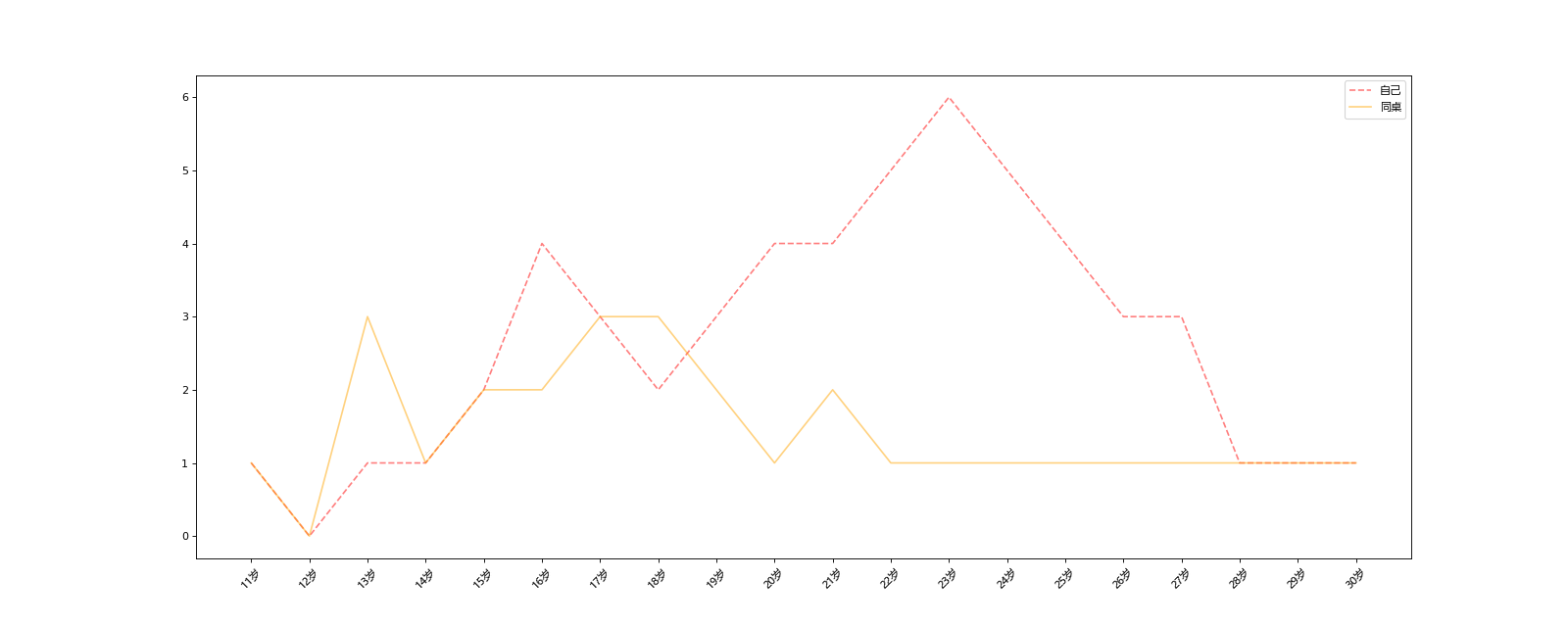数据科学库(HM）——第一节数据分析介绍&matplotlib1313-徐同学

数据分析

为什么要学数据分析？

1. python数据科学的基础(包含pandas、numpy、matplotlib等python库）
2. 机器学习课程的基础

1. 提出问题
2. 准备数据
3. 整理、分析数据
4. 获得结论
5. 成果可视化

matplotlib

matplotlib最流行的Python底层绘图库，主要做数据可视化图表,名字取材于MATLAB，模仿MATLAB构建

为什么学习matplotlib

• 能将数据进行可视化，更直观的呈现
• 是数据更加客观、更有说服力

matplotlib折线图的实现

#coding=utf-8
from matplotlib import pyplot as plt   #导入pyplot模块
#import matplotlib.pyplot asplt      另一种导入pyplot模块的方法

import random    #导入随机数模块
import matplotlib

plt.figure(figsize=(20,8),dpi=80)    #设置图片大小
'''1.figure图形图标的意思，在这里指的是我们绘制的图
2.通过实例化一个figure并且传递参数，能够在后台自动使用该figure实例
3.在图像模糊的时候可以传入dpi参数，让图像更加清晰'''

#设置显示中文字体
from matplotlib import font_manager
my_font = font_manager.FontProperties(fname=r"D:/anaconda/Lib/site-packages/matplotlib/mpl-data/fonts/ttf/msyh.ttc")

x=range(120)      #在x轴的位置，是一个可迭代的对象,生成[0,119]范围内的120个整数
y=[random.randint(20,35)for i in range(120)]  #random.randint(20,35):生成范围在（20，35）之间的随机数整数
#x轴和y轴的数据一起组成了所有要绘制的坐标点

plt.plot(x,y)    #传入x,y通过plot绘制出折线图

#设置x轴上字符串的刻度
_x_ticks=["10点{}分" .format(i) for i in x if i<60]+["11点{}分" .format(i-60) for i in x if i>60]
#x轴表示10点-12点每一分钟的时刻数

plt.xticks(x[::5],_x_ticks[::5],rotation=45,FontProperties=my_font)   #rotation设置旋转的角度
#设置步长（数字和字符串一一对应），数据的长度一样

#添加描述信息
plt.xlabel("时间",FontProperties=my_font)
plt.ylabel("温度 单位（°C）",FontProperties=my_font)
plt.title("十点到十二点每分钟的气温变化情况",FontProperties=my_font)
#显示中文的地方都需要传入FontProperties参数

plt.savefig("./t1.png")   #保存图片在当前目录下

plt.show()  #展示图形练习题：

a = [1,0,1,1,2,4,3,2,3,4,4,5,6,5,4,3,3,1,1,1]，b=[1,0,3,1,2,2,3,3,2,1 ,2,1,1,1,1,1,1,1,1,1

y轴表示个数

x轴表示岁数,比如11,12岁一直到30岁等

from matplotlib import pyplot as plt
import matplotlib

#设置显示中文字体
from matplotlib import font_manager
my_font = font_manager.FontProperties(fname=r"D:/anaconda/Lib/site-packages/matplotlib/mpl-data/fonts/ttf/msyh.ttc")

plt.figure(figsize=(20,8),dpi=80)    #设置图片大小

a = [1,0,1,1,2,4,3,2,3,4,4,5,6,5,4,3,3,1,1,1]
b = [1,0,3,1,2,2,3,3,2,1 ,2,1,1,1,1,1,1,1,1,1]

x=range(11,31)
plt.plot(x,a,label="自己",linestyle="--",color="red",alpha=0.5)
plt.plot(x,b,label="同桌",linestyle="-",color="orange",alpha=0.5)
#->通过label指定显示的图例内容

_x_ticks=["{}岁" .format(i) for i in x ]
#x轴表示11-30岁的年龄段

plt.xticks(x,_x_ticks,rotation=45,FontProperties=my_font)   #rotation设置旋转的角度

plt.legend(prop=my_font,loc="best")
#->通过prop指定图例的字体
#->通过loc指定图例的位置，默认右上角

plt.show()评论 抢沙发觉得文章有用就打赏一下文章作者

支付宝扫一扫打赏微信扫一扫打赏Vieu3.3主题Q Q 登 录